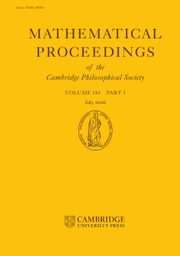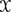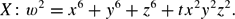Home
Hostname: page-component-99c86f546-4k54s Total loading time: 0.187 Render date: 2021-12-03T20:30:09.109Z Has data issue: true Feature Flags: { "shouldUseShareProductTool": true, "shouldUseHypothesis": true, "isUnsiloEnabled": true, "metricsAbstractViews": false, "figures": true, "newCiteModal": false, "newCitedByModal": true, "newEcommerce": true, "newUsageEvents": true }Mathematical Proceedings of the Cambridge Philosophical Society

# On the arithmetic of a family of degree - two K3 surfaces

Published online by Cambridge University Press:  27 March 2018

## Abstract

Let ℙ denote the weighted projective space with weights (1, 1, 1, 3) over the rationals, with coordinates x, y, z and w; let$\mathcal{X}$ be the generic element of the family of surfaces in ℙ given by\begin{equation*} X\colon w^2=x^6+y^6+z^6+tx^2y^2z^2. \end{equation*}
The surface$\mathcal{X}$ is a K3 surface over the function field ℚ(t). In this paper, we explicitly compute the geometric Picard lattice of$\mathcal{X}$, together with its Galois module structure, as well as derive more results on the arithmetic of$\mathcal{X}$ and other elements of the family X.

Type
Research Article

## Access options

Get access to the full version of this content by using one of the access options below. (Log in options will check for institutional or personal access. Content may require purchase if you do not have access.)

## References

[BCFNW17] Bouyer, F., Costa, E., Festi, D., Nicholls, C. and West, M.. Accompanying MAGMA code. http://www.staff.uni-mainz.de/dfesti/AWS2015Magma.txt (2017).Google Scholar
[BCP97] Bosma, W., Cannon, J. and Playoust, C. The Magma algebra system. I. The user language. J. Symbolic Comput. 24 (3-4) (1997), 235265. Computational algebra and number theory (London, 1993).10.1006/jsco.1996.0125CrossRefGoogle Scholar
[BHPVdV04] Barth, W. P., Hulek, K., Peters, C. A. M. and Van de Ven, A. Compact complex surfaces volume 4 of Ergeb. Math. Grenzgeb. 3. Folge. A Series of Modern Surveys in Mathematics [Results in Mathematics and Related Areas. 3rd Series. A Series of Modern Surveys in Mathematics]. (Springer-Verlag, Berlin, second edition, 2004).Google Scholar
[BT00] Bogomolov, F. A. and Tschinkel, Yu. Density of rational points on elliptic K3 surfaces. Asian J. Math. 4 (2) (2000), 351368.10.4310/AJM.2000.v4.n2.a6CrossRefGoogle Scholar
[Cha14] Charles, F. On the Picard number of K3 surfaces over number fields. Algebra Number Theory 8 (1) (2014), 117.10.2140/ant.2014.8.1CrossRefGoogle Scholar
[CS99] Conway, J. H. and Sloane, N. J. A. Sphere packings, lattices and groups. Grundlehren Math Wiss vol. 290 [Fundamental Principles of Mathematical Sciences]. (Springer-Verlag, New York, third edition, 1999). With additional contributions by Bannai, E., Borcherds, R. E., Leech, J., Norton, S. P., Odlyzko, A. M., Parker, R. A., Queen, L. and Venkov, B. B.Google Scholar
[Dol96] Dolgachev, I. V. Mirror symmetry for lattice polarised K3 surfaces. J. Math. Sci. 81 (3) (1996), 25992630. Algebraic geometry, 4.10.1007/BF02362332CrossRefGoogle Scholar
[EJ08] Elsenhans, A.-S. and Jahnel, J. K3 surfaces of Picard rank one and degree two. In Algorithmic number theory Lecture Notes in Comput. Sci. vol. 5011 (Springer, Berlin, 2008), pp. 212225.10.1007/978-3-540-79456-1_14CrossRefGoogle Scholar
[EJ11] Elsenhans, A.-S. and Jahnel, J. The Picard group of a K3 surface and its reduction modulo p. Algebra Number Theory 5 (8) (2011), 10271040.10.2140/ant.2011.5.1027CrossRefGoogle Scholar
[Fes16] Festi, D. Topics in the arithmetic of del Pezzo and K3 surfaces. PhD. thesis. Universiteit Leiden (2016).Google Scholar
[Har77] Hartshorne, R. Algebraic geometry Graduate Texts in Math. No. 52. (Springer-Verlag, New York-Heidelberg, 1977).10.1007/978-1-4757-3849-0CrossRefGoogle Scholar
[HKT13] Hassett, B., Kresch, A. and Tschinkel, Y. Effective computation of Picard groups and Brauer–Manin obstructions of degree two K3 surfaces over number fields. Rendiconti del Circolo Matematico di Palermo 62 (1) (2013), 137151.10.1007/s12215-013-0116-8CrossRefGoogle Scholar
[HS00] Hindry, M. and Silverman, J. H. Diophantine geometry: an introduction Graduate Texts in Math. vol. 201 (Springer-Verlag, New York, 2000).10.1007/978-1-4612-1210-2CrossRefGoogle Scholar
[Huy16] Huybrechts, D. Lectures on K3 surfaces Camb. Stud. Adv. Math. vol. 158 (Cambridge University Press, Cambridge, 2016).10.1017/CBO9781316594193CrossRefGoogle Scholar
[Ino78] Inose, H. Defining equations of singular K3 surfaces and a notion of isogeny. In Proceedings of the International Symposium on Algebraic Geometry (Kyoto Univ., Kyoto, 1977) (Kinokuniya Book Store, Tokyo, 1978), pp. 495–502.Google Scholar
[KT04] Kresch, A. and Tschinkel, Y. On the arithmetic of del Pezzo surfaces of degree 2. Proc. London Math. Soc. (3) 89 (3) (2004), 545569.10.1112/S002461150401490XCrossRefGoogle Scholar
[MP12] Maulik, D. and Poonen, B. Néron-Severi groups under specialisation. Duke Math. J. 161 (11) (2012), 21672206.10.1215/00127094-1699490CrossRefGoogle Scholar
[Mum70] Mumford, D. Abelian varieties. Tata Institute of Fundamental Research Studies in Mathematics, No. 5. Published for the Tata Institute of Fundamental Research, Bombay (Oxford University Press, London, 1970).Google Scholar
[Nik80a] Nikulin, V. V. Integer symmetric bilinear forms and some of their geometric applications. Math USSR-Izv 14 (1) (1980), 103167.10.1070/IM1980v014n01ABEH001060CrossRefGoogle Scholar
[Nik80b] Nikulin, V. V. Integral symmetric bilinear forms and some of their applications. Mathematics of the USSR-Izvestiya, 14 (1) (1980), 103.10.1070/IM1980v014n01ABEH001060CrossRefGoogle Scholar
[PTvL15] Poonen, B., Testa, D. and Luijk, R. van Computing Néron-Severi groups and cycle class groups. Compositio. Math. 151 (4) (2015), 713734.10.1112/S0010437X14007878CrossRefGoogle Scholar
[SS10] Schütt, M. and Shioda, T. Elliptic surfaces. In Algebraic geometry in East Asia–-Seoul 2008 Adv. Stud. Pure Math. vol. 60 (Math. Soc. Japan, Tokyo, 2010), pp. 51160.Google Scholar
[ST10] Stoll, M. and Testa, D. The surface parametrising cuboids. arXiv:1009.0388 (2010).Google Scholar
[VAV11] Várilly–Alvarado, A. and Viray, B. Failure of the Hasse principle for Enriques surfaces. Adv. Math. 226 (6) (2011), 48844901.10.1016/j.aim.2010.12.020CrossRefGoogle Scholar
[vL07] van Luijk, R. An elliptic K3 surface associated to Heron triangles. J. Number Theory 123 (1) (2007), 92119.10.1016/j.jnt.2006.06.006CrossRefGoogle Scholar
1
Cited by

# Send article to Kindle

Note you can select to send to either the @free.kindle.com or @kindle.com variations. ‘@free.kindle.com’ emails are free but can only be sent to your device when it is connected to wi-fi. ‘@kindle.com’ emails can be delivered even when you are not connected to wi-fi, but note that service fees apply.

Find out more about the Kindle Personal Document Service.

On the arithmetic of a family of degree - two K3 surfaces
Available formats
×

# Send article to Dropbox

To send this article to your Dropbox account, please select one or more formats and confirm that you agree to abide by our usage policies. If this is the first time you use this feature, you will be asked to authorise Cambridge Core to connect with your <service> account. Find out more about sending content to Dropbox.

On the arithmetic of a family of degree - two K3 surfaces
Available formats
×

# Send article to Google Drive

To send this article to your Google Drive account, please select one or more formats and confirm that you agree to abide by our usage policies. If this is the first time you use this feature, you will be asked to authorise Cambridge Core to connect with your <service> account. Find out more about sending content to Google Drive.

On the arithmetic of a family of degree - two K3 surfaces
Available formats
×
×